# Setup for 5 Player a Game of Food Chain Magnate

Originally generated on 1/11/2019 11:13:51 AM
A direct link to this setup is http://www.VentersConsulting.com/BoardgameHelpers/FoodChainMagnateGenerator.aspx?LoadGame=\$2a\$04\$zYWkgbOHavcxTVNGCGcaxe

## Player Setup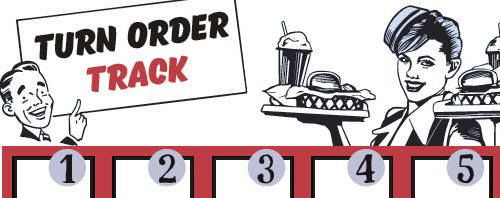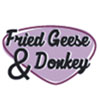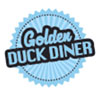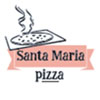## Other Setup

Bank starts with \$250.00
Number of 1x Employee cards used = 3

## Map (Dehydration Land)

Click here to view Map Tile Lettering Key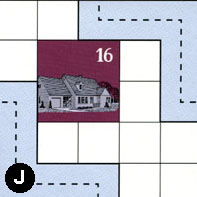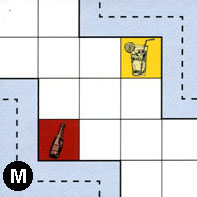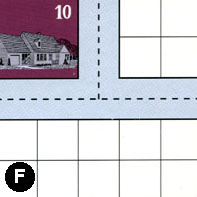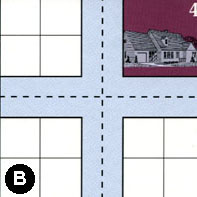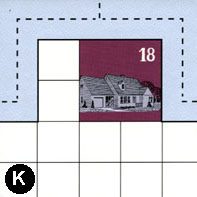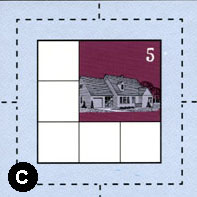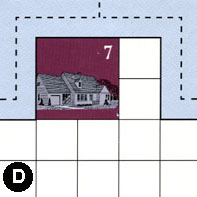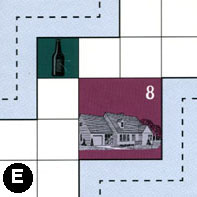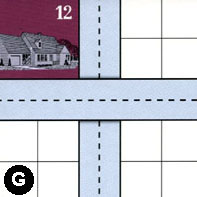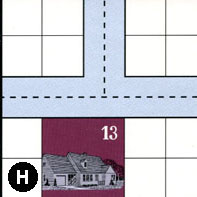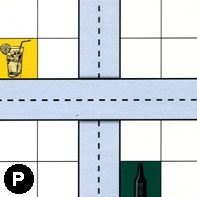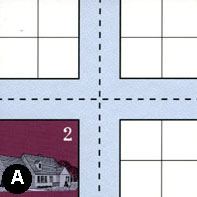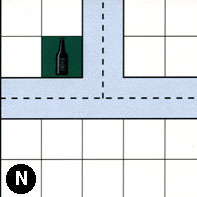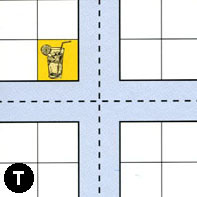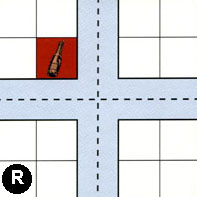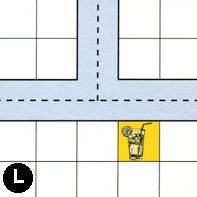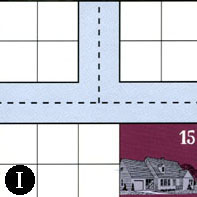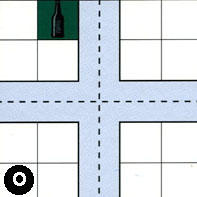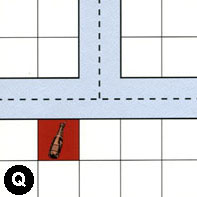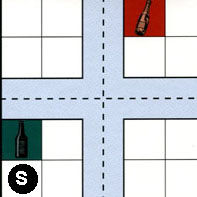### Map Stats

Map Option = Dehydration Land
Total Number of Tiles = 20
Total Number of Starting Houses = 11
Total Number of Beer Spots = 5
Total Number of Soda Spots = 4
Total Number of Lemonade Spots = 4
Total Number of Drink Spots = 13
Number of independent path systems = 3
Number of independent neighborhoods = 18

Path System 1
Size = Small
Number of Tiles = 2
Number of Paths = 2
Contains Loop(s) = No
Number of Starting Houses = 2
Starting Houses Pct of Map (%) = 18
Number of Beer Spots = 0
Number of Soda Spots = 0
Number of Lemonade Spots = 1
Number of Drink Spots = 1
Drink Spots Pct of Map (%) = 8
Tile Ids = J, M
Paths = JWS, MEN What do these mean?
Starting House Ids = 0, 16
Path System 2
Size = Medium
Number of Tiles = 18
Number of Paths = 68
Contains Loop(s) = No
Number of Starting Houses = 10
Starting Houses Pct of Map (%) = 91
Number of Beer Spots = 5
Number of Soda Spots = 3
Number of Lemonade Spots = 3
Number of Drink Spots = 11
Drink Spots Pct of Map (%) = 85
Tile Ids = A, B, C, D, E, G, H, I, J, K, L, N, O, P, Q, R, S, T
Paths = JEN, CWN, DWE, EWN, NWN, TWE, RWS, HWE, KWE, KWN, KNE, HWN, HNE, RWN, RNE, SWS, SWN, SNE, QWN, TWN, RNS, RES, SNS, SES, QNE, QWE, TNE, NNE, OWN, ONE, OWE, NWE, OWS, IWE, LWN, LNE, LWE, IWN, AWN, PWE, ANE, DWN, DNE, CNE, PNS, CWE, CWS, CNS, CES, AWE, INE, ONS, OES, AWS, ANS, AES, TES, GNS, BWN, BNE, BWE, BWS, BNS, BES, TWS, TNS, SWE, RWE What do these mean?
Starting House Ids = 0, 2, 4, 5, 7, 12, 13, 15, 16, 18
Path System 3
Size = Medium
Number of Tiles = 4
Number of Paths = 6
Contains Loop(s) = No
Number of Starting Houses = 5
Starting Houses Pct of Map (%) = 45
Number of Beer Spots = 0
Number of Soda Spots = 1
Number of Lemonade Spots = 0
Number of Drink Spots = 1
Drink Spots Pct of Map (%) = 8
Tile Ids = E, F, G, M
Paths = MWS, FWN, EES, GWE, FWE, FNE What do these mean?
Starting House Ids = 0, 8, 10, 12, 13

Neighborhood 1
Total Size = Huge
Number of Total Spaces = 77
Number of Empty Spaces (for new houses & gardens) = 57
Number of Beer Spots = 2
Number of Soda Spots = 1
Number of Lemonade Spots = 1
Number of Drink Spots = 4
Number of Starting Houses = 4
Starting Houses Pct of Map (%) = 36
Tile Ids = D, E, F, G, J, M, N, T
Starting House Ids = 7, 8, 10, 16
Neighborhood 2
Total Size = Small
Number of Total Spaces = 4
Number of Empty Spaces (for new houses & gardens) = 4
Number of Beer Spots = 0
Number of Soda Spots = 0
Number of Lemonade Spots = 0
Number of Drink Spots = 0
Number of Starting Houses = 0
Starting Houses Pct of Map (%) = 0
Tile Ids = F
Neighborhood 3
Total Size = Medium
Number of Total Spaces = 22
Number of Empty Spaces (for new houses & gardens) = 14
Number of Beer Spots = 0
Number of Soda Spots = 0
Number of Lemonade Spots = 0
Number of Drink Spots = 0
Number of Starting Houses = 2
Starting Houses Pct of Map (%) = 18
Tile Ids = B, F, G
Starting House Ids = 4, 12
Neighborhood 4
Total Size = Large
Number of Total Spaces = 50
Number of Empty Spaces (for new houses & gardens) = 42
Number of Beer Spots = 0
Number of Soda Spots = 0
Number of Lemonade Spots = 0
Number of Drink Spots = 0
Number of Starting Houses = 2
Starting Houses Pct of Map (%) = 18
Tile Ids = B, G, H, K, R, T
Starting House Ids = 13, 18
Neighborhood 5
Total Size = Small
Number of Total Spaces = 4
Number of Empty Spaces (for new houses & gardens) = 4
Number of Beer Spots = 0
Number of Soda Spots = 0
Number of Lemonade Spots = 0
Number of Drink Spots = 0
Number of Starting Houses = 0
Starting Houses Pct of Map (%) = 0
Tile Ids = H
Neighborhood 6
Total Size = Medium
Number of Total Spaces = 9
Number of Empty Spaces (for new houses & gardens) = 5
Number of Beer Spots = 0
Number of Soda Spots = 0
Number of Lemonade Spots = 0
Number of Drink Spots = 0
Number of Starting Houses = 1
Starting Houses Pct of Map (%) = 9
Tile Ids = C
Starting House Ids = 5
Neighborhood 7
Total Size = Medium
Number of Total Spaces = 8
Number of Empty Spaces (for new houses & gardens) = 7
Number of Beer Spots = 0
Number of Soda Spots = 1
Number of Lemonade Spots = 0
Number of Drink Spots = 1
Number of Starting Houses = 0
Starting Houses Pct of Map (%) = 0
Tile Ids = H, R
Neighborhood 8
Total Size = Small
Number of Total Spaces = 4
Number of Empty Spaces (for new houses & gardens) = 3
Number of Beer Spots = 1
Number of Soda Spots = 0
Number of Lemonade Spots = 0
Number of Drink Spots = 1
Number of Starting Houses = 0
Starting Houses Pct of Map (%) = 0
Tile Ids = P
Neighborhood 9
Total Size = Medium
Number of Total Spaces = 8
Number of Empty Spaces (for new houses & gardens) = 8
Number of Beer Spots = 0
Number of Soda Spots = 0
Number of Lemonade Spots = 0
Number of Drink Spots = 0
Number of Starting Houses = 0
Starting Houses Pct of Map (%) = 0
Tile Ids = A, P
Neighborhood 10
Total Size = Large
Number of Total Spaces = 26
Number of Empty Spaces (for new houses & gardens) = 22
Number of Beer Spots = 0
Number of Soda Spots = 0
Number of Lemonade Spots = 0
Number of Drink Spots = 0
Number of Starting Houses = 1
Starting Houses Pct of Map (%) = 9
Tile Ids = A, I, N, O
Starting House Ids = 2
Neighborhood 11
Total Size = Large
Number of Total Spaces = 26
Number of Empty Spaces (for new houses & gardens) = 24
Number of Beer Spots = 0
Number of Soda Spots = 0
Number of Lemonade Spots = 2
Number of Drink Spots = 2
Number of Starting Houses = 0
Starting Houses Pct of Map (%) = 0
Tile Ids = A, I, L, P
Neighborhood 12
Total Size = Large
Number of Total Spaces = 26
Number of Empty Spaces (for new houses & gardens) = 24
Number of Beer Spots = 1
Number of Soda Spots = 1
Number of Lemonade Spots = 0
Number of Drink Spots = 2
Number of Starting Houses = 0
Starting Houses Pct of Map (%) = 0
Tile Ids = N, O, Q, T
Neighborhood 13
Total Size = Medium
Number of Total Spaces = 16
Number of Empty Spaces (for new houses & gardens) = 15
Number of Beer Spots = 0
Number of Soda Spots = 0
Number of Lemonade Spots = 1
Number of Drink Spots = 1
Number of Starting Houses = 0
Starting Houses Pct of Map (%) = 0
Tile Ids = Q, R, S, T
Neighborhood 14
Total Size = Medium
Number of Total Spaces = 8
Number of Empty Spaces (for new houses & gardens) = 7
Number of Beer Spots = 1
Number of Soda Spots = 0
Number of Lemonade Spots = 0
Number of Drink Spots = 1
Number of Starting Houses = 0
Starting Houses Pct of Map (%) = 0
Tile Ids = R, S
Neighborhood 15
Total Size = Small
Number of Total Spaces = 4
Number of Empty Spaces (for new houses & gardens) = 4
Number of Beer Spots = 0
Number of Soda Spots = 0
Number of Lemonade Spots = 0
Number of Drink Spots = 0
Number of Starting Houses = 0
Starting Houses Pct of Map (%) = 0
Tile Ids = L
Neighborhood 16
Total Size = Medium
Number of Total Spaces = 18
Number of Empty Spaces (for new houses & gardens) = 14
Number of Beer Spots = 0
Number of Soda Spots = 0
Number of Lemonade Spots = 0
Number of Drink Spots = 0
Number of Starting Houses = 1
Starting Houses Pct of Map (%) = 9
Tile Ids = I, L, O
Starting House Ids = 15
Neighborhood 17
Total Size = Medium
Number of Total Spaces = 8
Number of Empty Spaces (for new houses & gardens) = 7
Number of Beer Spots = 0
Number of Soda Spots = 1
Number of Lemonade Spots = 0
Number of Drink Spots = 1
Number of Starting Houses = 0
Starting Houses Pct of Map (%) = 0
Tile Ids = Q, S
Neighborhood 18
Total Size = Small
Number of Total Spaces = 4
Number of Empty Spaces (for new houses & gardens) = 4
Number of Beer Spots = 0
Number of Soda Spots = 0
Number of Lemonade Spots = 0
Number of Drink Spots = 0
Number of Starting Houses = 0
Starting Houses Pct of Map (%) = 0
Tile Ids = S

v8.3.2